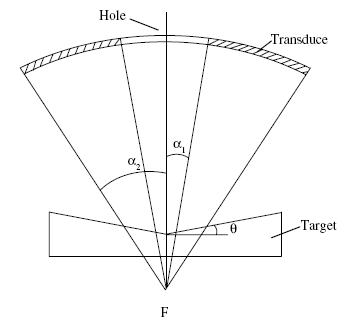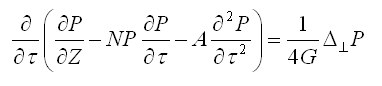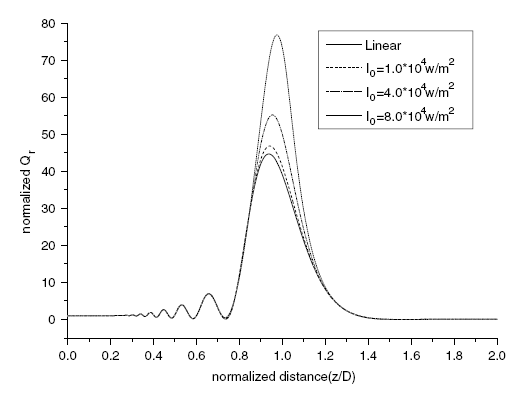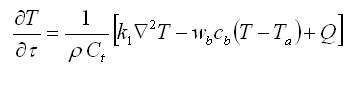High Intensity Focused Ultrasound Physics, Instrumentation, & Medical Applications
 Introduction Physics Instrumentation Medical Applications References
 The Physics of High Intensity Focused Ultrasound Ultrasound waves are acoustic pressure waves that have a frequency range that begins at the limit of human hearing, 20 kHz, and extends well into the megahertz range.  When propagated into human tissue, the pressure waves are either reflected or absorbed.  Reflected ultrasound waves are the basis for the commonly used ultrasound imaging technique in the body.  HIFU therapy differs from ultrasound imaging in that the waves are of a higher intensity (power) and are focused at a single point. The mechanism of HIFU therapeutic action takes two forms: conversion of mechanical energy into heat and mechanical cavitation of pressure waves in tissues.  By focusing the ultrasound waves at a particular spot in the body, the energy converted to heat causes the tissue to heat up and kill the cells in the tissue.  In this way, HIFU treatment is similar to other ablation techniques such as radio frequency ablation and cryoablation.  The other therapeutic action of HIFU is through cavitation, which causes the tissue to vibrate and mechanically stress the cells, causing them to also die.  Since mechanical cavitation is unpredictable, it is avoided in developing instruments for HIFU therapeutic application . Important aspects of the physics of HIFU include the relationship between the axial radiation force and acoustic power, acoustic propagation, the time-rate temperature change during HIFU radiation, the spatial and intensity dependence of heat deposition from HIFU, and finite element based methods of HIFU simulation . Force v. Power The relationship between the axial radiation force (F) and acoustic power (P) of a given HIFU transducer is important since this relationship is directly tied in to the temperature at the irradiation site.  The relationship is given by the following radiation force balance equation :Where the quantities are:A schematic of the system described by the force balance equation is shown here :Acoustic Propagation The acoustic propagation  of HIFU is described by the Khokhlov–Zabolotkaya–Kuznetsov (KZK) equation given here in cylindrical coordinates:Where the quantities are:The solution to this equation, P, is the sound pressure and is a Fourier series expansion, is a function of the radius of the source, focal length of the source, and retarded time. Heat Deposition Heat deposition is a function of a constant (alpha) proportional to the sound attenuation coefficient, the frequency, and fundamental frequency of the wave, as well as the ultrasound intensity I:The heat deposition as a function of the source distance at different intensities was computer simulated and is given here :Temperature Distribution The time-rate temperature distribution in biological tissue is given by the Pennes heat transfer equation:Where the quantities are:The temperature distribution has been measured empirically using a thermocouple-based system and compared to a linear and nonlinear predictive model of temperature distribution in porcine tissue .  A plot of the difference in the model distribution and actual distribution is shown here:Created by Austin Moy BME 240: Intro to Clinical Medicine Biomedical Engineering University of California, Irvine Last Updated: June 11, 2008